# Need of Feature Scaling in Machine Learning

Data collection and pre-processing of the raw form of data is the ultimate necessity. Big organizations in data science and machine learning domains record many attributes/properties to avoid losing critical information. Every attribute has its properties and valid ranges in which it can lie. For example, the speed of a motorbike can be in the range of 0–200 KM/h, but the speed of cars can be in the range of 0–400 KM/h. Machine learning or deep learning models expect these ranges to be on the same scale to decide the importance of these properties without any bias.

In this article, we will learn about one of the essential topics used in scaling different attributes for machine learning: Normalization and Standardization. Even among machine learning professionals, the confusion for the selection between normalization and standardization persists. Through this article, we will try to clear this confusion forever.

1. What is Normalization?
2. Why do we need scaling (normalization or standardization)?
3. What are different normalization techniques?
4. What is Standardization?
5. When to normalize and when to standardize?

In machine learning, an individual property that can be measured or characteristic of an observed phenomenon is a feature. Based on the availability of essential and independent observations, we train our model with a combination of input features. For example, suppose we want to train a machine learning model to predict the flat price. We can efficiently train our model with the size of the flat as our feature. But including the locality of the flat in our input features set will improve the performance of our model. Hence, we use various observable and independent features to make our model more sure about the predictions.As the features are different, so the ranges of their numerical values would also be different. The process of scaling all the features into the same definite range is known as Normalization.

But shouldn’t we ask Why? Why scale the features? Why not directly use features and train the model?

Let’s go through one example to answer this question, which will open the mathematical angle supporting normalization or standardization.

Suppose we have to make a machine learning model learn the function, Y = mX + c. We have been given the dataset ( Input and Output). During the learning process, the machine will start from randomly selected values ( or hard-coded manual values*) for m and c. Theniteratively reduce the error between the predicted value of Y ( i.e., Y^) and the actual value of Y. Our overall goal is to minimize this error function.

Let’s choose our error function, which can also be called as cost function, MSE. The formulae for MSE are given in the below equation, where n is the number of training samples.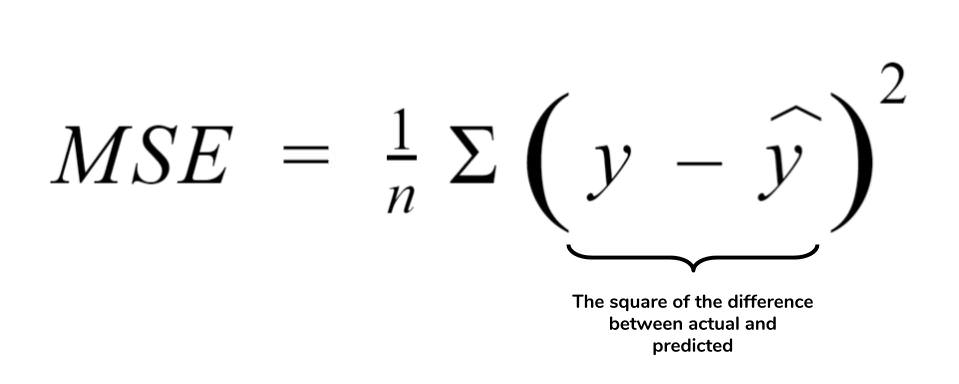As Y is a function that depends upon two variables, m, and c, hence cost function will also depend on these two variables. In the GIF below, there is one dimension of the Cost function, and the rest two dimensions can be considered as m and c.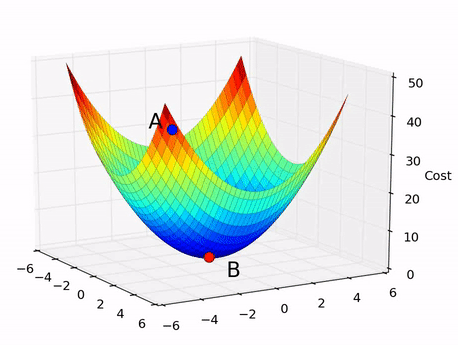At the start, suppose we are at position A (Shown in GIF above) and reaching position B is our ultimate goal as that is the minima of the cost function. For that, the machine will tweak the values of m and c.

But the machine can take infinite values for m and c if it selects these values randomly at each step. We use optimizers to help the machine choose the following values of m and c to reach the minima quickly. Let’s choose gradient descent as our optimizer to learn the function Y = m*X + c. In gradient descent, we update the value of any parameter using the below formulae.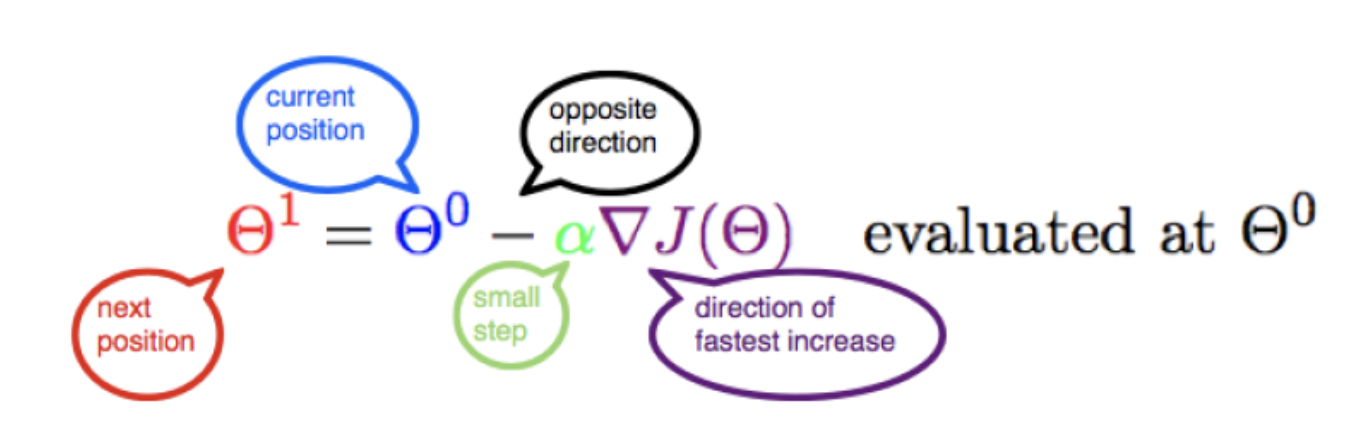Source: Coursehero

let’s say we updated the value of m and c by using the above formulae, then new m and c will be :

#### m = m-(α * ẟm) c = c-(α * ẟc)

Let’s calculate m and c. Prediction error can be represented in the equation as error = (Y^ — Y)

Cost function :Now let’s calculate the partial derivative of this cost function concerning two variables, m, and c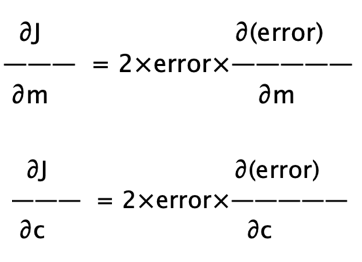Also,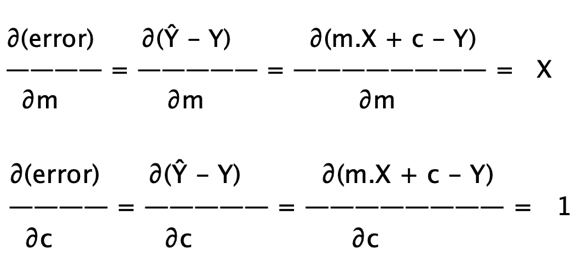After combining the equations and putting everything in the gradient descent formulae,

#### c1 = c0 — α * error

The presence of feature value X in the above update formula will affect the step size of the gradient descent. If the features are in different ranges, it will cause different step sizes for every feature. In the image below, let’s say x1 = c, and x2 = m. To ensure the functionality of the gradient descent moves smoothly towards the minima and steps for gradient descent get updated at the same rate for every feature, we scale the data before feeding it to the model.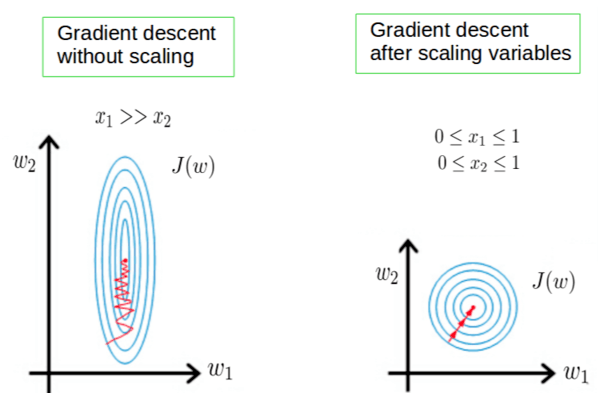Source: Medium

Some machine learning algorithms are susceptible to normalization or standardization, and some are insensitive to it. Algorithms like SVM, K-NN, K-means, Neural Networks, or Deep-learning are susceptible to normalization/standardization. These algorithms use the spatial relationships ( Space dependent relations) present among the data samples.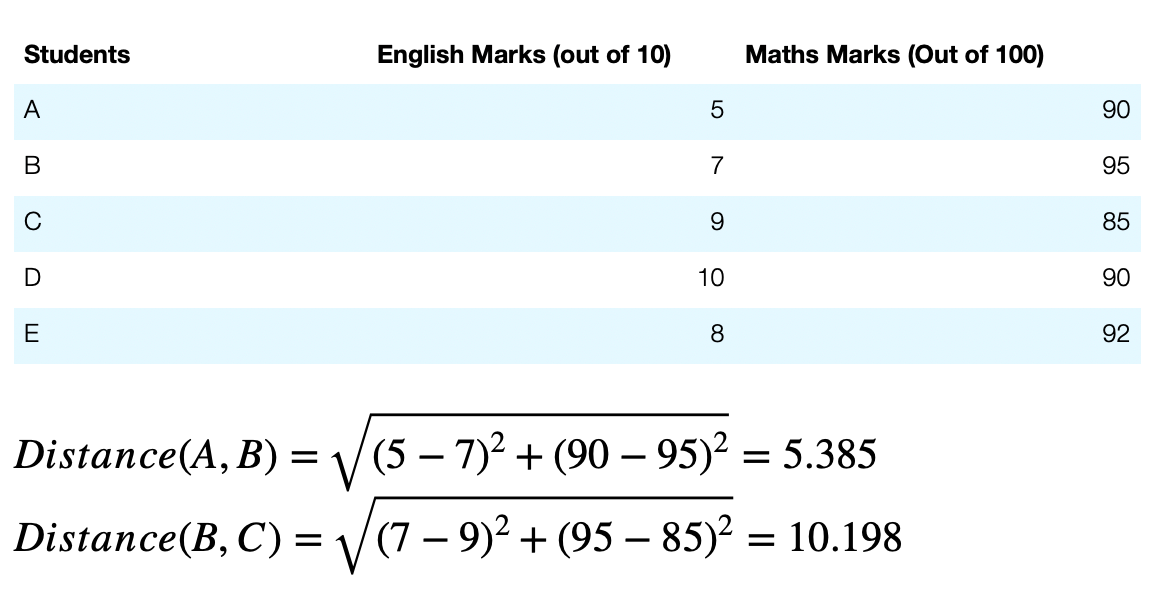Let’s use the scaling technique and use the percentage of marks instead of direct marks.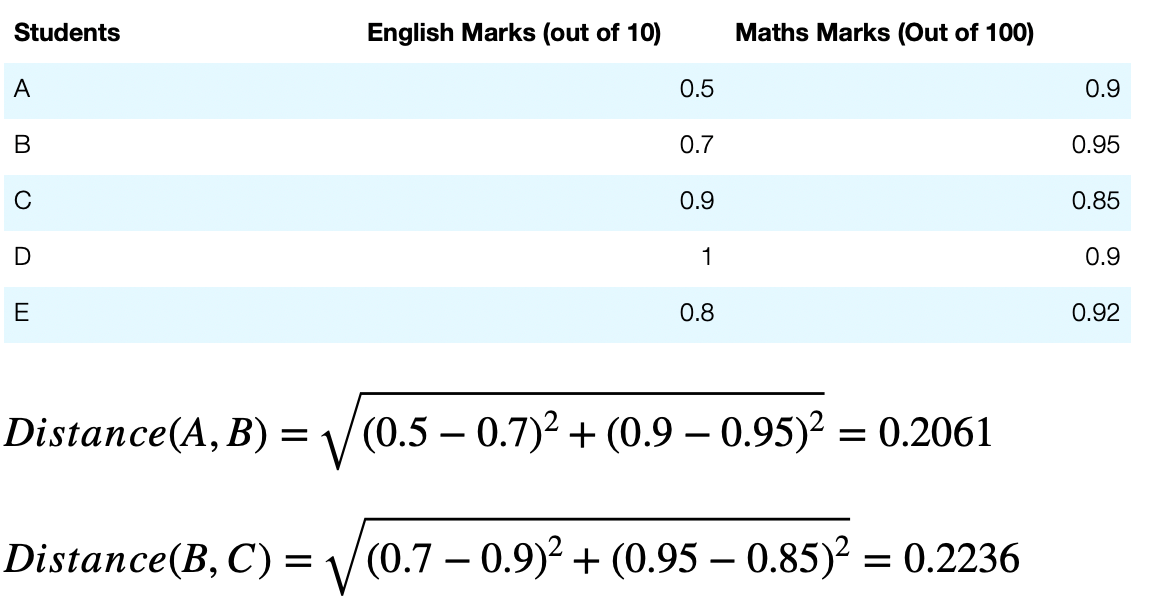The scaled distances are closer and can be compared easily.

Algorithms like Decision trees, Random forests,s or other tree-based algorithms are insensitive to normalization or standardization as they are being applied on every feature individually and not influenced by any other feature.

So the two reasons that support the need for scaling are:

1. Scaling the features makes the flow of gradient descent smooth and helps algorithms quickly reach the minima of the cost function.
2. Without scaling features, the algorithm may be biased towards the feature which has values higher in magnitude. Hence we scale features that bring every feature in the same range, and the model uses every feature wisely.

#### Popular Scaling techniques:

1.Min-Max Normalization
In range [0, 1]In the range of [-1, 1]In range [a, b ] (Generalised)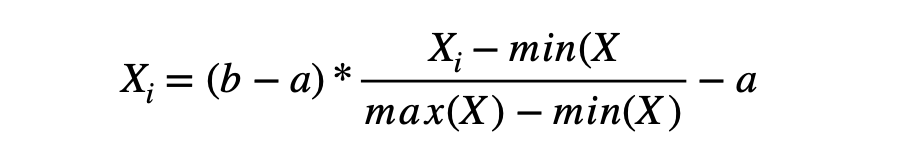2. Logistic Normalization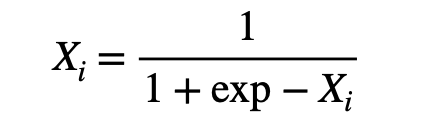Standardization

Standardization is another scaling technique in which we transform the feature such that the transformed features will have mean (μ) = 0 and standard deviation (σ) = 1.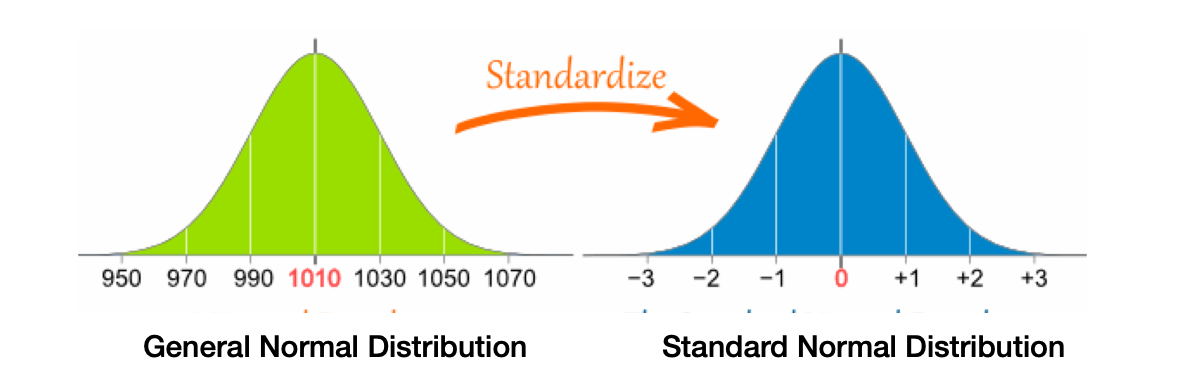The formula to standardize the features in data samples is :This scaling technique is also known as Z-Score normalization or Z-mean normalization. Unlike normalization, standardization techniques are not much affected by the presence of outliers (Think!).

Now, we know two different scaling techniques. But sometimes, knowing more or having more options brings another challenge of choice. So we have a new question for us,

When to Normalize and When to Standardize?

Let’s learn a bit more, which will end this doubt as well.

#### Normalization would be beneficial when,

1. Data samples are NOT normally distributed.
2. Dataset is clean or free from outliers.
3. The dataset covers all the corner ( Minimum or Maximum ) ranges of features.
4. They are used for the algorithms like Neural Networks, K-NN, K-means.

#### Standardization would be beneficial when,

1. Data samples are from a normal distribution. This is not always to be true, but most effectiveness will be observed when it will happen.
2. The dataset contains outliers that can affect the min/max calculations.

#### Summary :

1. Scaling features helps optimization algorithms to reach the minima of cost function quickly.
2. Scaling features restrict models from being biased towards features having higher/lower magnitude values.
3. Normalization and Standardization are two scaling techniques.
4. With gaussian( normal) distributed data samples, standardization works perfectly.

#### Possible Interview Question on Normalization

1. What is data normalization, and why do we need it?
2. Do we need to normalize the output/target variable as well?
3. What is standardization? When is standardization preferred?
4. If we do not scale the variables, why will the model become biased?
5. Why standardization seems to be better as per the real-life scenarios?

#### Conclusion

In this article, we saw the need to scale different attributes in Machine Learning. Data Science and Machine learning fields expect all the features or attributes to be present on the same scale to decide the importance of those features without any biases. We have shown using two different examples how scaling helps in building the machine learning models. In the last, we also discussed one of the main challenges, even for machine learning professions, that when to use which scaling techniques. We hope you have enjoyed the article.

#### Enjoy Learning! Enjoy Scaling! Enjoy Algorithms!

Get well-designed application and interview centirc content on ds-algorithms, machine learning, system design and oops. Content will be delivered weekly.